Középiskolai Matematikai és Fizikai Lapok
Informatika rovattal
 Már regisztráltál? Új vendég vagy?# Exercises and problems in Physics May 1999

## New experimental problem:

m. 207. Investigate how the volume of an inflated, initially approximately spherical balloon changes as the balloon is gradually pulled under water using a thread.

## New exercises:

FGy. 3254. There is half a metre of oil with a density of 800 kg/m3 atop half a metre of water in a barrel. A prism with a length of 1 m and a cross-section of 1 dm2 floats in this liquid. The bottom 10 cm of this prism is made of aluminium with a density of 2700 kg/m3, the rest is made of pinewood with a density of 500 kg/m3. The prism is slowly and cautiously lifted out from the liquid. How much work do we do and how much work does the upthrust do? (The prism remains vertical all the time.)

FGy. 3255. There is 10 g of oxygen in a cylinder closed by a piston. This gas goes through the equilibrium states described by the formula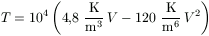while its volume increases from the initial 10 litres to 25 litres. What are the lowest and highest pressures and temperatures of the gas during this process?

FGy. 3256. There is one point-like body with a charge of 6.10-8 C and a mass of 2 g at rest in each vertex of an equilateral triangle with 50 cm sides. What are the electric field strength and the potential at the centre of the triangle? What is the initial acceleration of the bodies when their fastenings are simultaneously released, and what final speed do they reach? (All other forces should be neglected.)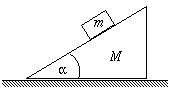FGy. 3257. A prism of mass M=3 kg forming a slope of inclination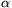can slide without friction on a horizontal table. A rectangular body of mass m=1 kg is placed onto this slope, where it can slide down without friction, while the prism moves with some acceleration. At what angleis the acceleration of the prism maximum?

## New problems:

FF. 3258. A flea goes up a flight of stairs jumping from edge to edge on the stairs. When can he get up with less work: when he takes the stairs one by one or two by two? In which case does he arrive upstairs sooner?

FF. 3259. A ship can increase its speed using a built-in pump that sucks in a mass m of water per unit time through an opening in the ship's bottom, and ejaculating it backwards at speed c with respect to the ship. What is the maximum speed that the ship can reach if the drag is proportional to the square of its speed: Fk=B.v2?

FF. 3260. A metal sphere can be charged to charge Q1 using a belt generator. When it touches another, uncharged metal sphere, charge Q2 is passed over. Continuing this procedure - i.e. touching the first sphere to the belt generator and then to the second sphere -, what is the maximum charge that can accumulate on the second sphere?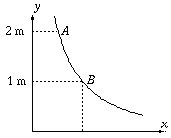FF. 3261. A point-like body with a mass of 0.1 kg starts with zero initial speed from point A of a hyperbolic slope of equation y=1/x, as shown in the figure. What is the pressing force in point B if friction is negligible?

FF. 3262. There is helium gas in a horizontal cylinder with heat insulating walls, closed by a piston. The piston of mass m can move without friction in the cylinder. The atmospheric pressure is pa; the temperature of the helium is initially T0, its volume is V0. Releasing the fastening of the piston, the atmospheric pressure suddenly compresses the gas, heating it up to temperature T. What is the maximum speed of the piston during this process? (Data: m=100 kg, pa=105 Pa, V0=1 m3, T0=300 K, T=600 K.)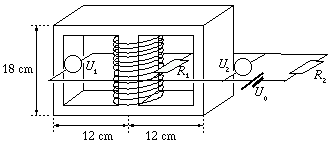FN. 3263. There is a coil of 3600 turns in the central part of the iron core shown in the figure. The cross-section of the coil is 20 cm2 here and 5 cm2 elsewhere. The relative permeability of iron is 4600. What pd. do the voltmeters read, while the current flowing in the coil increases uniformly from zero to 10 A in 0.1 s? (The internal resistance of the battery is negligible. U0=18 V, R1=3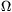, R2=2.)